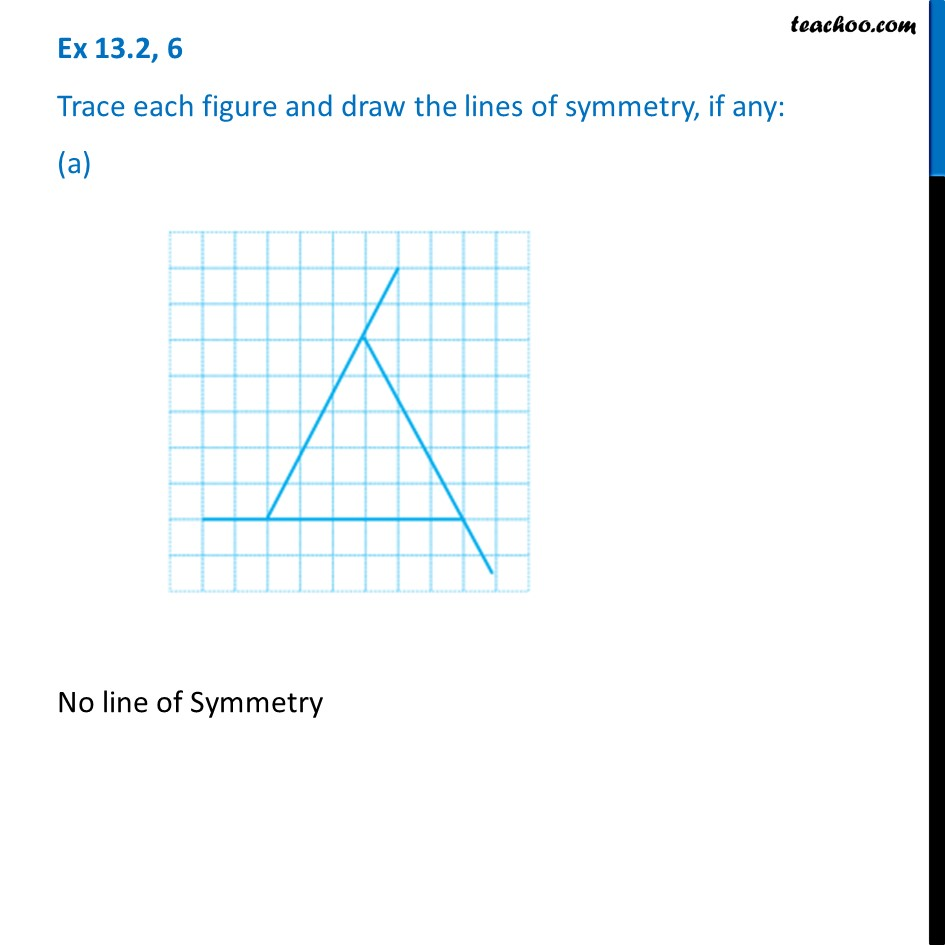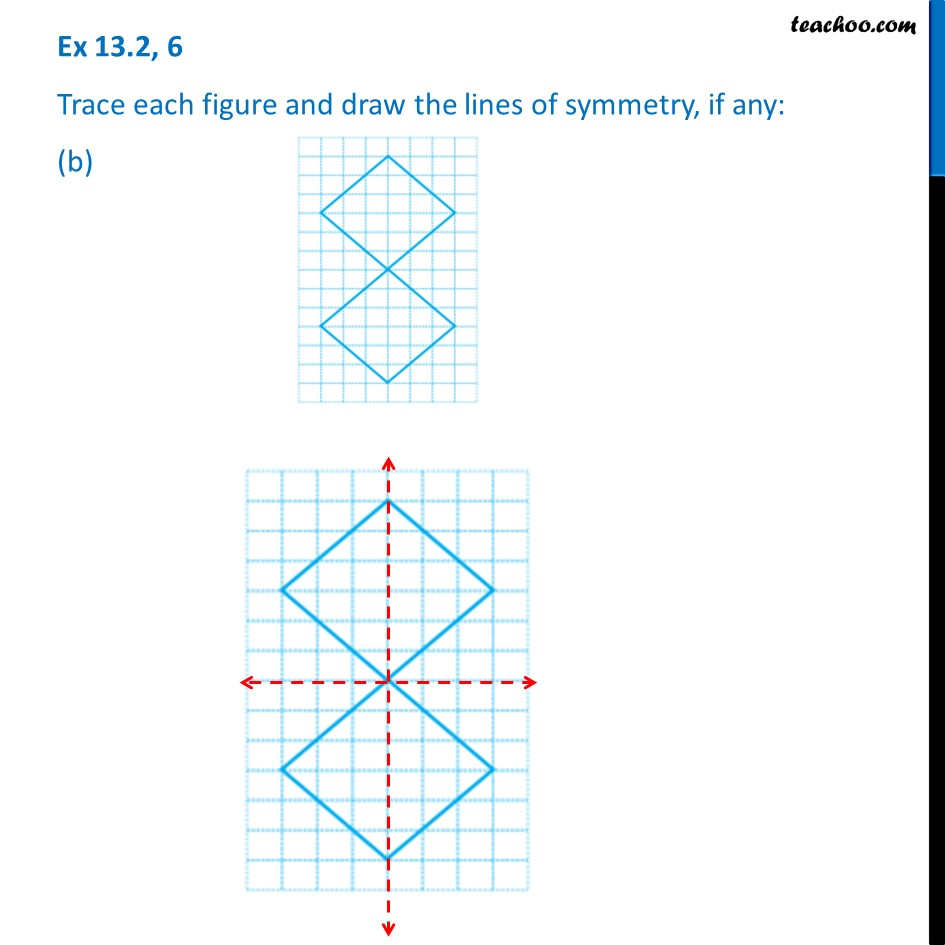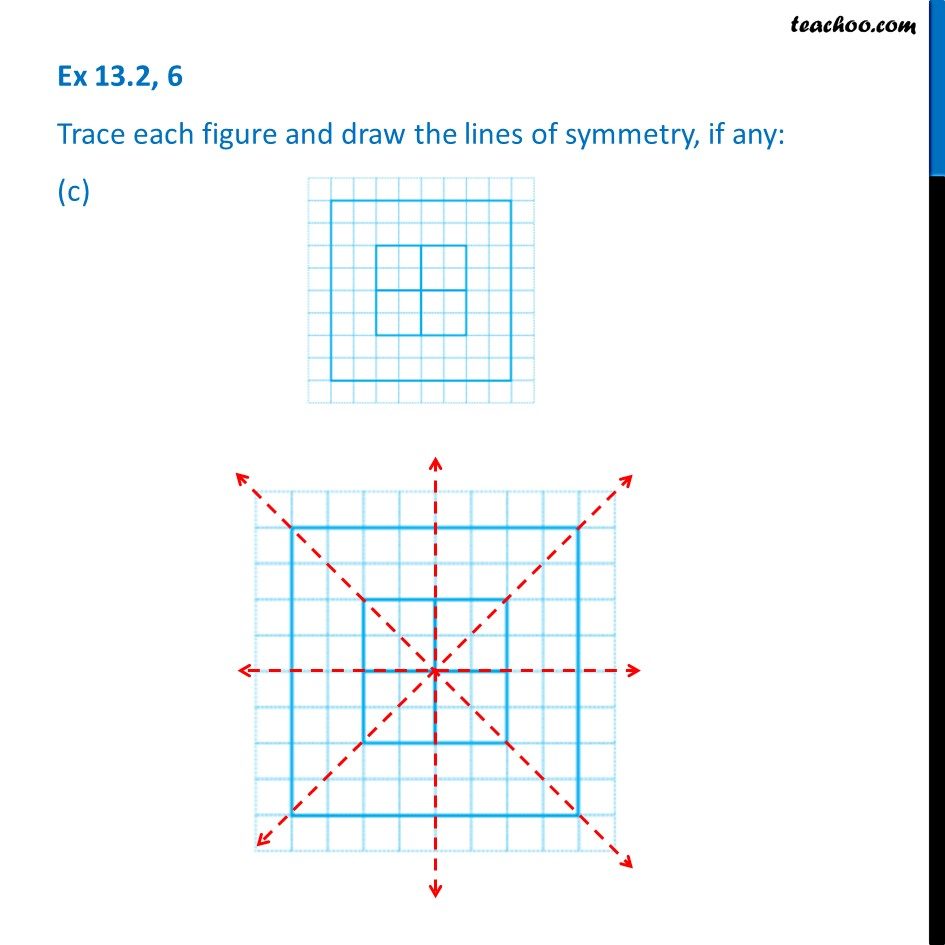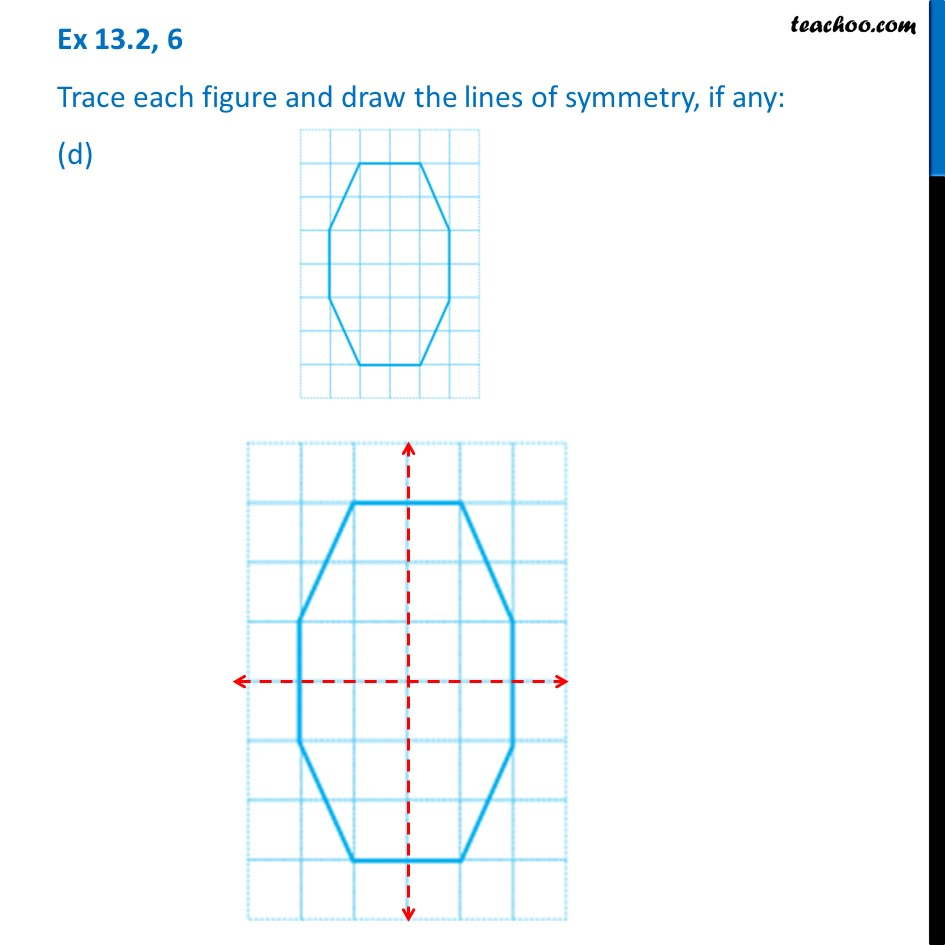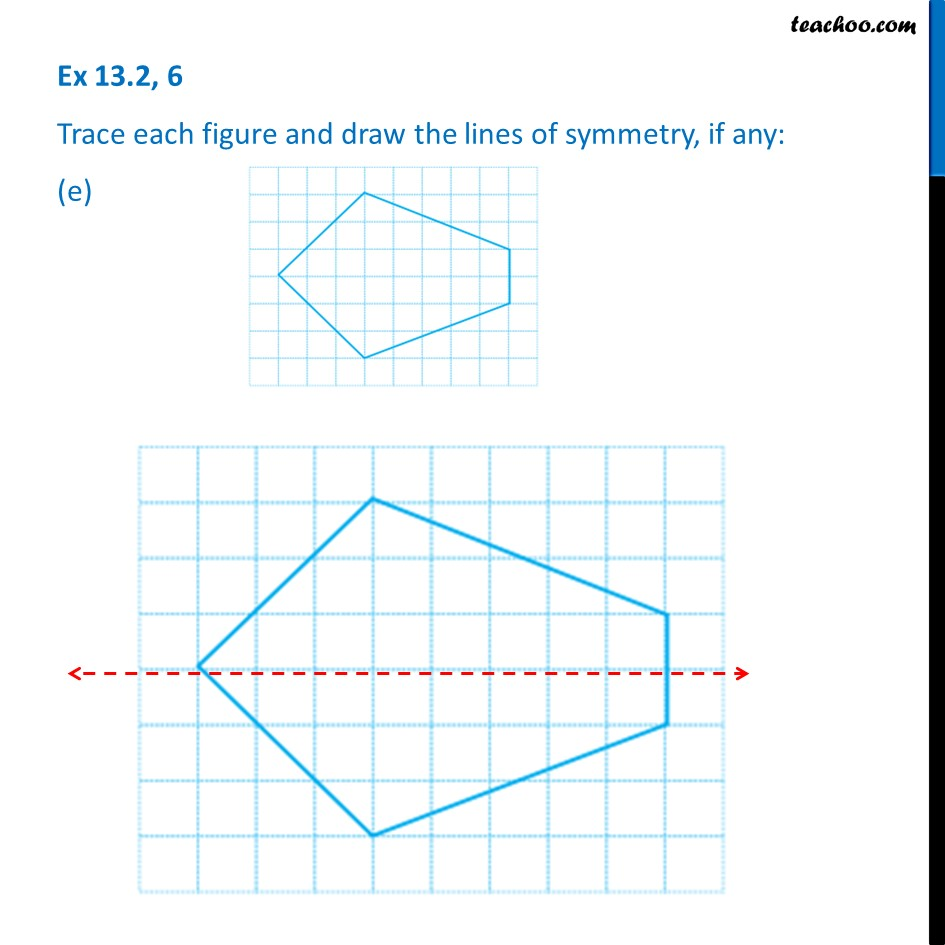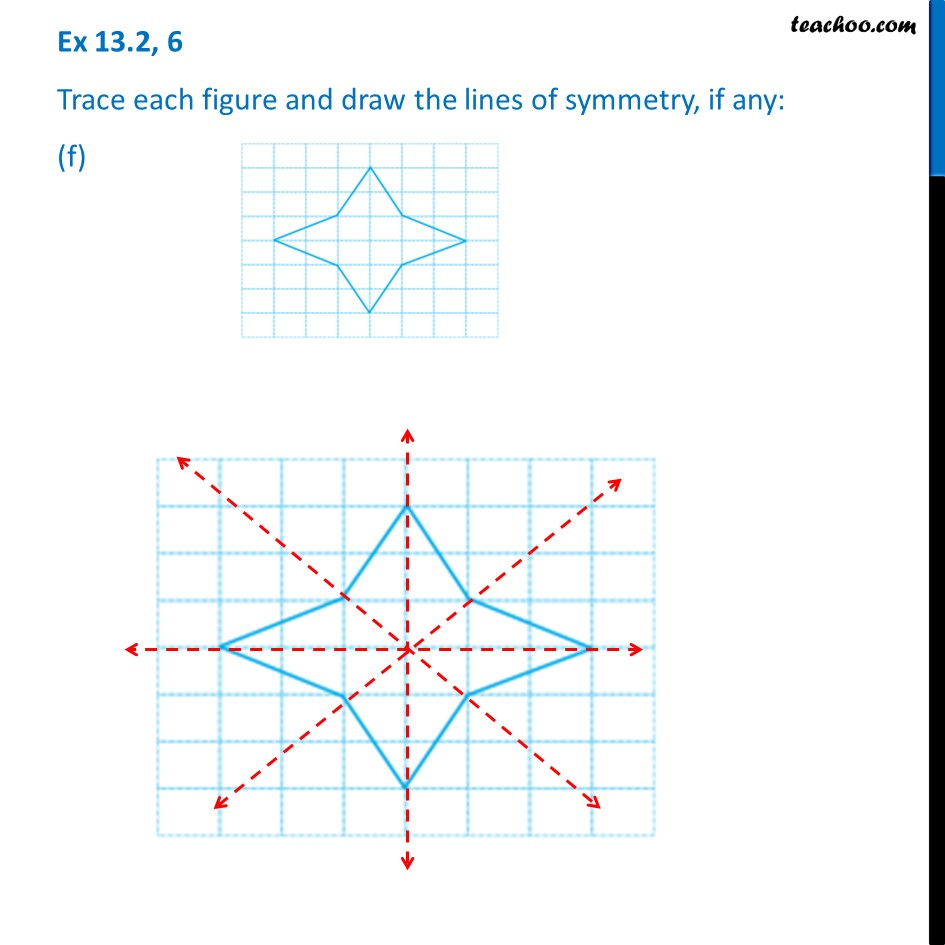1. Chapter 13 Class 6 Symmetry
2. Serial order wise
3. Ex 13.2

Transcript

Ex 13.2, 6 Trace each figure and draw the lines of symmetry, if any: (a)No line of Symmetry Ex 13.2, 6 Trace each figure and draw the lines of symmetry, if any: (b)Ex 13.2, 6 Trace each figure and draw the lines of symmetry, if any: (c)Ex 13.2, 6 Trace each figure and draw the lines of symmetry, if any: (d)Ex 13.2, 6 Trace each figure and draw the lines of symmetry, if any: (e)Ex 13.2, 6 Trace each figure and draw the lines of symmetry, if any: (f)

Ex 13.2

Chapter 13 Class 6 Symmetry
Serial order wise

About the AuthorDavneet Singh
Davneet Singh is a graduate from Indian Institute of Technology, Kanpur. He has been teaching from the past 10 years. He provides courses for Maths and Science at Teachoo.